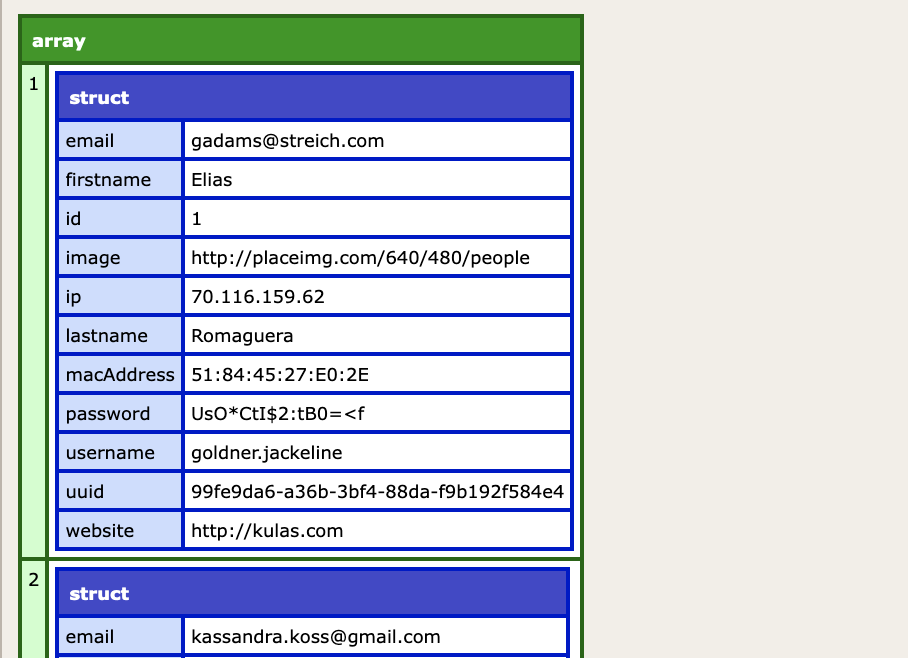Matthew J. Clemente

# TIL: Use arraySet to Initialize an Array of a Specific Size

Jul 27, 2022
3 minutes

I recently learned about the function `arraySet`. It's a niche function, to be sure, but I nevertheless found that it served a useful purpose when creating arrays.

To start, I'll just quote the docs, regarding what `arraySet` does:

In a one-dimensional array, sets the elements in a specified index range to a value. Useful for initializing an array after a call to arrayNew.

As the docs helpfully point out, the use/purpose of the function is array initialization right after the array is created. Let's demonstrate that functionality with a simple example using the member function syntax:

``example = ArrayNew(1).set(1,10,"hi");writeDump(example);// ["hi","hi","hi","hi","hi","hi","hi","hi","hi","hi"]``

For a minute, ignore that the example is pointless; it illustrates how `arraySet` can be used to immediately set the length of a new array to 10. That functionality is (believe it or not) useful!

## How is it useful?

The short answer is that `arraySet`, when combined with a mapping function, can be used to initialize an array of a specific size with a range of values. This is really handy for generating data when testing, putting together a demo, or if you just need some placeholder data while scaffolding an application.

Let's take a look at how this would work; here's an example generating an array of three random users:

``random_users = ArrayNew(1).set(1,3,0).map( () => getRandomUser() );function getRandomUser() {  var result = '';  cfhttp(    url="https://fakerapi.it/api/v1/users?_quantity=1",    result="result"  );  var response = deserializeJson(result.Filecontent);  return response.data;}// dump array with user datawriteDump(random_users);``

In this example, we're using `arraySet` to take a newly created empty array and set its length to three elements, with `0` as their initial value. The array's size is limited to three because 1) this is an example and 2) we want to be polite to the external API that we're using.

We then chain this three element array to a `map` function, replacing each element with the value returned from our `getRandomUser()` function. Here, we're using the Faker API to get some random user data. By the end, we can dump an array with data for three random users, which would look like this:Obviously you could get the same results using a loop, but I find this syntax preferable because it can be chained into a single command.

So, there you go - another tool that might come in handy, should the need arise.

#### Footnotes

1. If you're into playing code golf, you might be interested that Lucee enables you to write the example even more succinctly, using implicit array syntax `[].set(1,10,"hi")`. The fact that it errors in Adobe ColdFusion is probably a bug. ↩︎